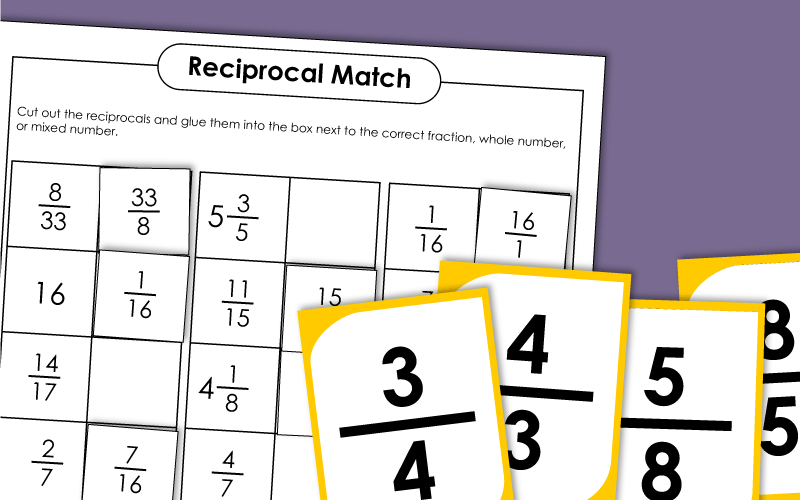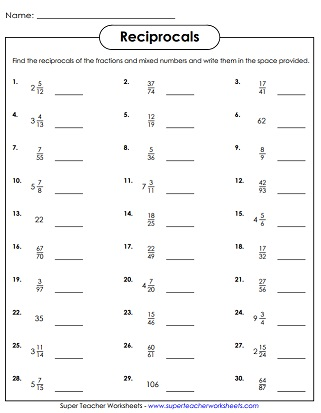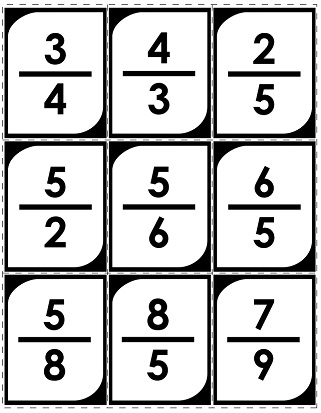# Reciprocal Fraction Worksheets

The worksheets on this page can be used to teach or review reciprocal fractions. (A reciprocal fractions is sometimes referred to as a multiplicative inverse.)This worksheet shows how to find the reciprocal of fractions and mixed numbers.
Part 1: Write the reciprocal for each fraction or mixed number.
Part 2: Multiply each fraction times its reciprocal to complete each equation.
Review basic reciprocals with this memory match card game. This version has only basic fractions,and does not include mixed numbers.
Learn about reciprocals with this memory match card game. This version has mixed numbers and whole numbers.
Cut out the fraction squares. Glue each one next to its reciprocal.

Fraction Worksheets

Full list of fraction topics. Includes simplification of fractions, ordering, comparing, and converting to decimals. There are also worksheets on adding, subtracting, multiplying, and dividing fractions.

Multiplying and Dividing Fractions

The worksheets on this page can be used to review fraction multiplication and division.

## Sample Worksheet ImagesMy Account
Site Information